Mathematical and Physical Journal
for High Schools
Issued by the MATFUND Foundation
 Already signed up? New to KöMaL?

# Exercises and problems in PhysicsSeptember 2002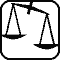## Experimental problem

M. 235. Determine the direction of the magnetic induction around a disk shaped magnet. First use some iron dust then a compass. (6 points)## Theoretical problems

It is allowed to send solutions for any number of problems, but final scores of students of grades 9-12 are computed from the 5 best score in each month. Final scores of students of grades 1-8 are computed from the 3 best scores in each month.

P. 3541. In a vessel filled with water to its half there is a closed, thin walled glass sphere. On the same table there also stand two glasses of equal size entirely filled with water. Pouring the contents of one of them into the vessel we see that the glass sphere sinks a bit deeper. If we pour the contents of the other glass into the vessel the glass sphere floats higher. How can that happen? (3 points)

P. 3542. How can the mass of a test tube be estimated with the help of a graduated cylinder and the side-piped vessel shown in the figure. (3 points)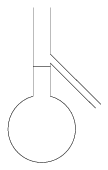P. 3543. Eve, the 176 cm tall model is looking at herself in a mirror hanging on the wall. The mirror is vertical and its height is 75 cm. Eve stands straight, her eyes are at a height of 168 cm from the floor. Can she see her eyes if she can just see her shoes? (3 points)

P. 3544. From the vessel shown in the figure the air is pumped out. The upper sphere is filled with water. If the lower sphere is cooled with liquid nitrogen, the water in the upper vessel freezes after some time. How can this phenomenon be explained? Is it possible for the water to freeze while boiling? (4 points)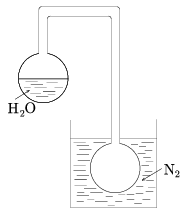P. 3545. Two spherical shaped equal sized glass fishbowls filled with water are standing on the table. In the middle of each fishbowl a small fish is swimming. How large and in what distance can they see each other? The refraction index of water is 1.33. (5 points)

P. 3546. A 1000 kg space ship with a 100 kg astronaut inside and a 100 kg satellite are floating in space at a 20 m distance from each other. The pilot kicks himself off the space ship to adjust an element of an aerial on the satellite. His velocity relative to the space ship is 1.1 m/s. He concludes the job in 130 seconds, and then he wants to return to the space ship in 100 seconds. At what velocity should he kick himself off the satellite to achieve this? (4 points)

P. 3547. Geostationary satellites are put into orbit as follows: first the satellite is in a low, Earth parking orbit, then by firing its engines for a short period an elliptic orbit is achieved with its close Earth point (perigee) on the parking orbit and the farthest from the Earth point(apogee) on the stationary orbit. When the satellite reaches the furthest point, it is put into a circular orbit by firing the engines again. Let us assume that the parking orbit is a circular orbit of an altitude of 200 km above Earth's surface. How long does it take for the satellite to reach the distance of the geostationary orbit? (5 points)

P. 3548. The resistance of the current consumers in the electric network seen in the figure is R1=300 $\displaystyle Omega$ and R2=700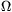. The electromotive force of the power source is U0=50 V, and its internal resistance is negligible. The capacity of the capacitor is C=50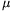F. Connecting the voltmeter between points A and B it shows an U1=14.5 V voltage.

a) What is the resistance of the voltmeter? How many electrons leave the negative coating of the capacitor during the measurement?

b) What would the voltmeter show connecting it between points B and D? What is the absolute and the relative error of this measurement?

c) At least how much the resistance of the voltmeter should be that connecting it between points B and D the relative error of the measurement is 1%. (5 points)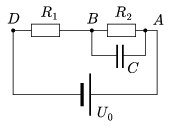P. 3549. An optical grating is lit with parallel light beams of 589 nm wavelength. On a screen at a 3 meter distance of the grating the distance of the zero order and first order diffraction maxima is 20 cm.

a) How many lines are there in the grating in one cm?

b) How far is the second order diffraction maximum from the first order one? (4 points)

P. 3550. In an Earth orbiting spaceship a floating laser pen of mass 50 g suddenly switches on, and emits light of 500 nm wavelength with a power of 3 mW for one hour.

a) How many photons are emitted during this period?

b) Estimate the velocity gained and the distance covered by the pen in the spaceship during this time. (5 points)